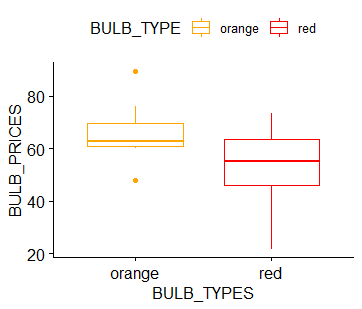# Mann Whitney U Test in R Programming

• Difficulty Level : Expert
• Last Updated : 28 Dec, 2021

A popular nonparametric(distribution-free) test to compare outcomes between two independent groups is the Mann Whitney U test. When comparing two independent samples, when the outcome is not normally distributed and the samples are small, a nonparametric test is appropriate. It is used to see the distribution difference between two independent variables on the basis of an ordinal(categorical variable having intrinsic an order or rank) dependent variable. It’s very much easy to perform this test in R programming.

### Implementation of Mann Whitney U Test in R Programming

Let’s say we have two kinds of bulbs say orange and red in our data and these are divided on the day to day base prices. So here the base prices are dependent variable on the two categories which are red and orange. So we will try and analyze that if we want to buy a red or orange color bulb which should we prefer on the basis of prices. If both the distributions are the same then this means that the null hypothesis (means no significant difference between the two) is true and we can buy any one of them and prices won’t matter. To understand the concept of the Mann Whitney U Test one needs to know what is the p-value. This value actually tells if we can reject our null hypothesis(0.5) or not. Now below is the implementation of the above example.

#### Approach

1.  Make a dataframe with two categorical variables in which one would be an ordinal type.
2. After this, check the summary of the non-ordinal categorical variable by loading a package dplyr and summarise() to get median values using median() and passing bulb_prices column, IQR-inter-quartile range, and count of both the groups i.e red and orange bulb.
3. Then look at the Boxplot and see the distribution of the data with the help of installing a package ggpubr and using the ggboxplot() and passing the columns as arguments in x and y and giving them color with help of palette and passing the color codes.
4. Then finally apply the function wilcox.test() to get the p-value.
5. If the p-value is found to be less than 0.5 then the null hypothesis will be rejected.
6. If we found the value to be greater than 0.5 then the null hypothesis will be accepted.
7. wilcox.test() function takes both categorical variables,dataframe as an argument, and gives us the hypothesis p-value.

## R

 `# R program to illustrate``# Mann Whitney U Test` `# Creating a small dataset``# Creating a vector of red bulb and orange prices``red_bulb <- ``c``(38.9, 61.2, 73.3, 21.8, 63.4, 64.6, 48.4, 48.8)``orange_bulb <- ``c``(47.8, 60, 63.4, 76, 89.4, 67.3, 61.3, 62.4)` `# Passing them in the columns``BULB_PRICE = ``c``(red_bulb, orange_bulb)``BULB_TYPE = ``rep``(``c``(``"red"``, ``"orange"``), each = 8)` `# Now creating a dataframe``DATASET <- ``data.frame``(BULB_TYPE, BULB_PRICE, stringsAsFactors = ``TRUE``)` `# printing the dataframe``DATASET` `# installing libraries to view summaries and``# boxplot of both orange and red color bulbs``install.packages``(``"dplyr"``)``install.packages``(``"ggpubr"``)` `# Summary of the data` `# loading the package``library``(dplyr)``group_by``(DATASET,BULB_TYPE) %>%``  ``summarise``(``    ``count = ``n``(),``    ``median = ``median``(BULB_PRICE, na.rm = ``TRUE``),``    ``IQR = ``IQR``(BULB_PRICE, na.rm = ``TRUE``))` `# loading package for boxplot``library``(``"ggpubr"``)``ggboxplot``(DATASET, x = ``"BULB_TYPE"``, y = ``"BULB_PRICE"``,``          ``color = ``"BULB_TYPE"``, palette = ``c``(``"#FFA500"``, ``"#FF0000"``),``          ``ylab = ``"BULB_PRICES"``, xlab = ``"BULB_TYPES"``)` `res <- ``wilcox.test``(BULB_PRICE~ BULB_TYPE,``                   ``data = DATASET,``                   ``exact = ``FALSE``)``res`

Output:

> DATASET

```     BULB_TYPE BULB_PRICE
1        red       38.9
2        red       61.2
3        red       73.3
4        red       21.8
5        red       63.4
6        red       64.6
7        red       48.4
8        red       48.8
9     orange       47.8
10    orange       60.0
11    orange       63.4
12    orange       76.0
13    orange       89.4
14    orange       67.3
15    orange       61.3
16    orange       62.4```

# summary of the data

```summarise()` ungrouping output (override with `.groups` argument)
# A tibble: 2 x 4
BULB_TYPE count median   IQR
<fct>     <int>  <dbl> <dbl>
1 orange        8   62.9   8.5
2 red           8   55    17.7```

# boxplot> res

```        Wilcoxon rank sum test with continuity correction

data:  BULB_PRICE by BULB_TYPE
W = 44.5, p-value = 0.2072
alternative hypothesis: true location shift is not equal to 0```

Explanation:

Here as we can see that the value of p is coming out to be 0.2072  which is far less than the null hypothesis(0.5). Due to which it will be rejected. And it can conclude that the distribution of prices over red and orange bulbs is not the same. Due to which it cannot say that if it is profitable to buy any one of the above bulbs is profitable.

My Personal Notes arrow_drop_up